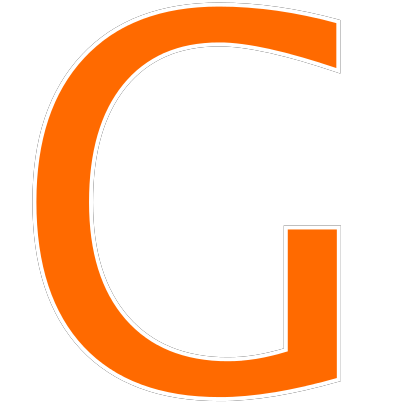# The Lock Has 3 Digit Code 291 Puzzle ANSWER (Solved)

The Lock Has 3 Digit Code 291, The Lock Has 3 Digit Code Puzzle Answer, 291 245 lock puzzle answer is the latest puzzle trending on the internet nowadays. The question is also being widely shared on WhatsApp, Facebook, Twitter, and Reddit. Many people from across the globe are trying to solve this puzzle and challenging their friends and family members to solve it correctly.

Here in this post, we are going to provide you the answer to the puzzle “The Lock Has 3 Digit Code 291“.

## The Lock Has 3 Digit Code 291 Answer

The Lock Has 3 Digit Code Puzzle Answer is 394

## The Lock Has 3 Digit Code 291 Puzzle Explanation

So as I have told you the answer to The Lock Has 3 Digit Code Puzzle is 394, so now let me show you how we came up with this answer.

As per the 4th Clue that says “nothing is correct”. So this means we have three numbers i.e. 5, 7 and 8 as not correct. Now let’s remove 5, 7 & 3 from all the rows.Now as per the 1st and 2nd clues i.e. One Number is Correct and in the Right Place and One Number is Correct but in the Wrong Place, we can see that number 2 is in the first position as well as its contradiction. So number 2 is not correct. Thereafter, now we can see that the number 4 is the correct one.We can also see number 4 in the 3rd row and the clue says two numbers are correct but in the wrong place. This makes 4 on the 2nd position as wrong as well as number 4 on the 1st position as wrong.Therefore Number 4 is in the third position of the code.

Now we have the 3rd code, so let us find the 1st and the 2nd code. Till now we have the following numbers removed from the clues. Have a look on the picture below:-Now lets check the first clue to find the next correct number. Clue 1 says One Number is Correct and in the Right Place. As you can see that 4 we got it in the 3rd place so 1 can not be the right number. Therefore now we have 9 remaining so 9 is our correct number and it has to be placed at the 2nd position.Now as per the 3rd Clue, it says Two Numbers are Correct but in the Wrong Place. As we have already got 9 as the correct number in the middle position. So 6 can not be the correct number in clue 3.

So number 3 is the correct number and it is placed in the first place.Therefore, the correct answer for The Lock Has 3 Digit Code is “394”